StatLect

# Precision matrix

The precision matrix of a random vector is the inverse of its covariance matrix.## Synonyms

The precision matrix is sometimes called concentration matrix.

## Definition

The following is a precise definition.

Definition Letbe arandom vector. Letbe its covariance matrix: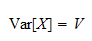Ifis invertible, then the precision matrix ofis thematrixdefined as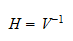Whenis a random variable (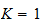), then the precision matrix becomes a scalar and it is equal to the reciprocal of the variance of. In this case, it is often denoted by the lowercase letter: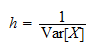and it is simply called the precision of.

Thus, in the univariate case precision is inversely proportional to variance: when variance tends to infinity, we have zero precision; on the contrary, when variance tends to zero, we have infinite precision.

## Precision matrix and normal densities

The joint probability density function of a multivariate normal random vector is often written in terms of its precision matrix.

Ifhas a multivariate normal distribution with meanand covariance matrix, then its joint probability density function is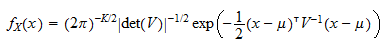By using the precision matrix, this can be written as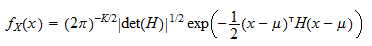because, by elementary properties of the determinant, we have that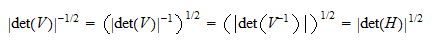In the univariate case, whenis a normal random variable with meanand variance, the density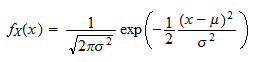becomes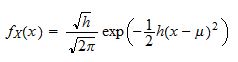Parametrizing a normal density in terms of its precision matrix often has significant advantages. For example, it can simplify the algebra of calculations involving normal densities. Or, when the values of a multivariate normal density need to be computed several times by numerical methods, employing the precision matrix can spare the computationally burdensome task of performing several matrix inversions to calculate.

## More details

You can read more details about covariance matrices in the lecture entitled Covariance matrix.